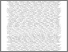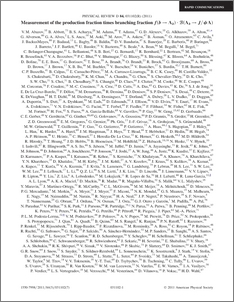# Measurement of the production fraction times branching fraction f(b --> Λ_b)B(Λ_b --> J/ψΛ)

Collaboration, D0 and Bertram, Iain and Borissov, Guennadi and Fox, Harald and Ross, Anthony and Williams, Mark and Ratoff, Peter (2011) Measurement of the production fraction times branching fraction f(b --> Λ_b)B(Λ_b --> J/ψΛ). Physical Review D, 84 (3). ISSN 1550-7998Preview
PDF
PhysRevD.84.031102.pdf - Published Version

## Abstract

The \Lambda_b(udb) baryon is observed in the decay \Lambda_b --> J/\psi \Lambda using 6.1 fb^{-1} of p\bar{p} collisions collected with the D0 detector at \sqrt{s}=1.96 TeV. The production fraction multiplied by the branching fraction for this decay relative to that for the decay B^0 --> J/\psi K^0_s is measured to be 0.345 \pm 0.034 (stat.) \pm 0.033 (syst.) \pm 0.003 (PDG). Using the world average value of f(b --> B^0)B(B^0 --> J/\psi K^0_s) = (1.74 \pm 0.08) \times 10^{-5}, we obtain f(b --> \Lambda_b)B(\Lambda_{b} --> J/\psi \Lambda) = (6.01 \pm 0.60 (stat.) \pm 0.58 (syst.) \pm 0.28 (PDG)) \times 10^{-5}. This measurement represents an improvement in precision by about a factor of three with respect to the current world average.

Item Type:
Journal Article
Journal or Publication Title:
Physical Review D